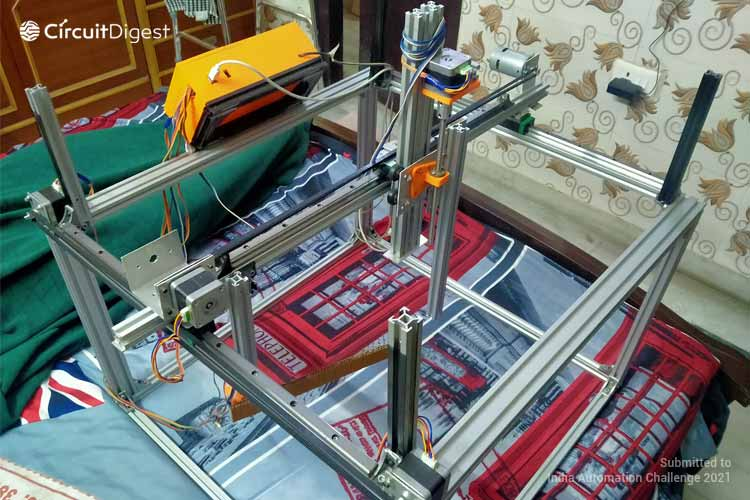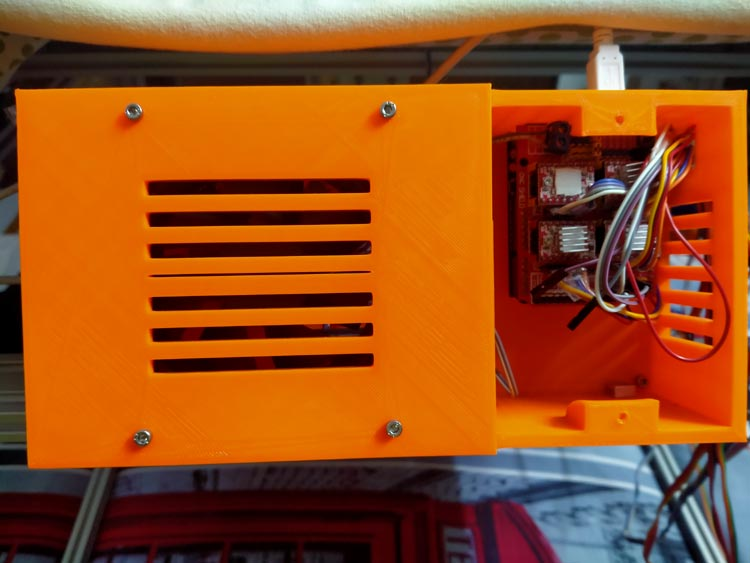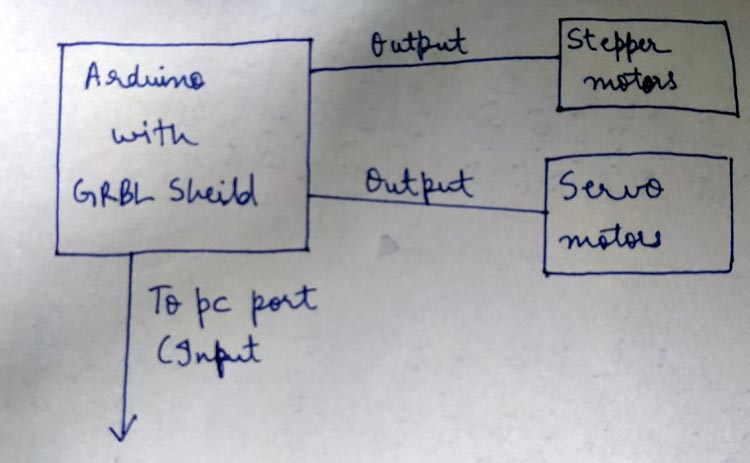1381 22
Kanishk Jain

## HealBot Semi Auto Surgical Machine

Problems faced- Due to growing road traffic, lack of doctors in many places and sometimes non...

### Project Used HardwareArduino UNO, GRBL Shield, Stepper motors and drivers, Servo motors, Gaming joystick, Aluminium Extrusions, GT2 timing belts, Nut bolts, threaded rod, POM Wheels, linear rails, etc.

### Project Used Software

Arduino IDE, Processing 3.0 IDE, Autodesk Fusion 360, Cura by Ultimaker.

### Project Hardware Software Selection

Hardware- All open electronics such as Arduino, GRBL shield are been used in this project to ensure the low cost and durability of this project. For robust structure and further solidification of the frame, aluminium extrusions are being used which are cheap and lightweight as well. Software- For Arduino simple Arduino software that is Arduino IDE is used and for controlling the machine java scripting on Processing 3.0 is used.

### Circuit DiagramThe circuit is quite simple just like wiring a CNC machine except servo are also connected for tool head support.

##### Code

``` ```

```import processing.sound.*; import processing.video.*; import processing.serial.*; import net.java.games.input.*; import org.gamecontrolplus.*; import org.gamecontrolplus.gui.*; import g4p_controls.*; import cc.arduino.*; import org.firmata.*; ControlDevice cont; ControlIO control; Arduino arduino; SoundFile sound; ControlButton aButton; ControlButton xButton; ControlButton yButton; ControlButton bButton; ControlButton lbButton; ControlButton rbButton; ControlHat hat; float thumb; float thumb1; Capture camera; int aint; int xint; int bint; int yint; int dval; int dval1; int dval2; int dval3; int lb; int rb; int pro = 0; int button = 0; int oldbutton = 0; PShape rectangle; void setup() {  size(1080, 720);  frameRate(30);  rectMode(CENTER);  camera = new Capture(this, "pipeline:autovideosrc");  camera.start();  //sound = new SoundFile(this, "data/screenshotsound.mp3");  //sound.play();  control = ControlIO.getInstance(this);  cont = control.getMatchedDevice("test3");  if (cont == null) {    println("dude its not moving");    System.exit(-1);  }  //println(Arduino.list());  arduino = new Arduino(this, Arduino.list(), 57600);  arduino.pinMode(11, Arduino.SERVO);  arduino.pinMode(10, Arduino.SERVO);  arduino.pinMode(2, Arduino.OUTPUT);  arduino.pinMode(5, Arduino.OUTPUT);  // arduino.pinMode(3, Arduino.OUTPUT);  //arduino.pinMode(4, Arduino.OUTPUT);  //arduino.pinMode(6, Arduino.OUTPUT);  //arduino.pinMode(7, Arduino.OUTPUT);  //arduino.pinMode(11, Arduino.OUTPUT);  //arduino.pinMode(12, Arduino.OUTPUT);  //arduino.pinMode(13, Arduino.OUTPUT); } public void getUserInput() {  thumb = map(cont.getSlider("Cut").getValue(), -1, 1, 0, 180);  thumb1 = map(cont.getSlider("rotation").getValue(), -1, 1, 180, 0);  aButton = cont.getButton("led");  xButton = cont.getButton("led1");  yButton = cont.getButton("ybutton");  bButton = cont.getButton("bbutton");  lbButton = cont.getButton("fast");  rbButton = cont.getButton("slow");  hat = cont.getHat("led2");  dval = 1;  dval1 = 1;  dval2 = 1;  dval3 = 1;  lb = 1;  if (lbButton.pressed() == true) {    lb = 0;  }  if (hat.down() == true) {    dval = 0;  }```

``` ```